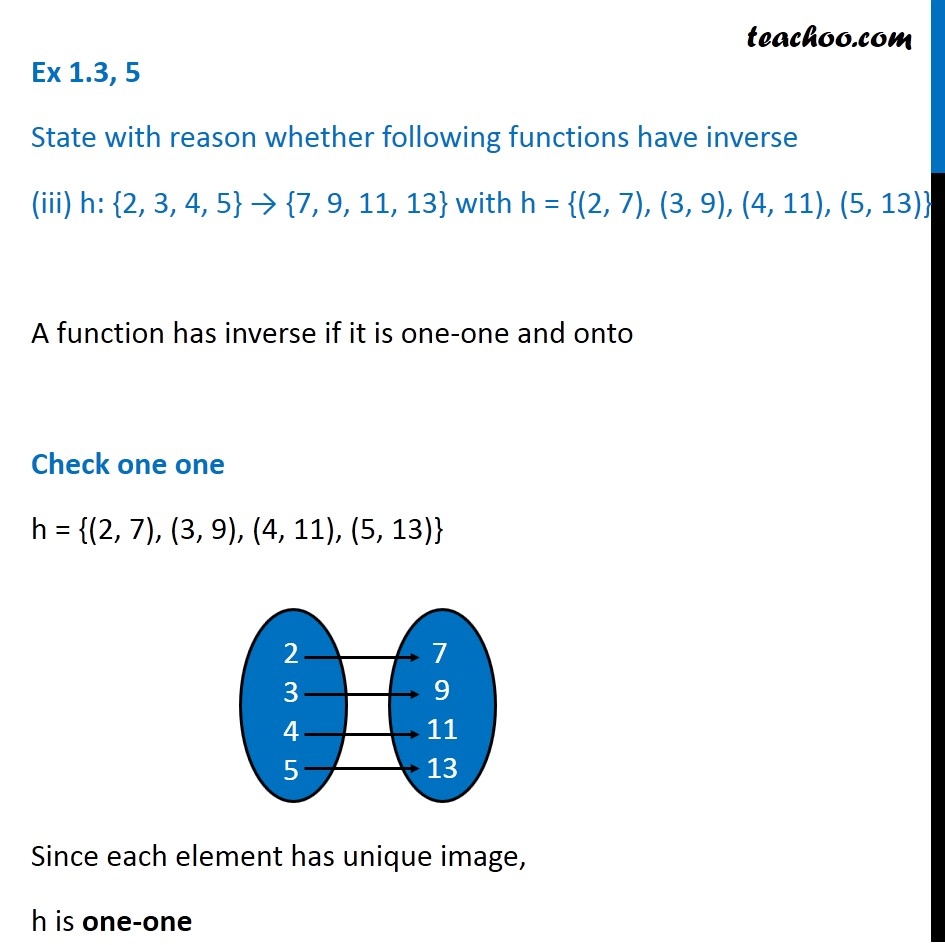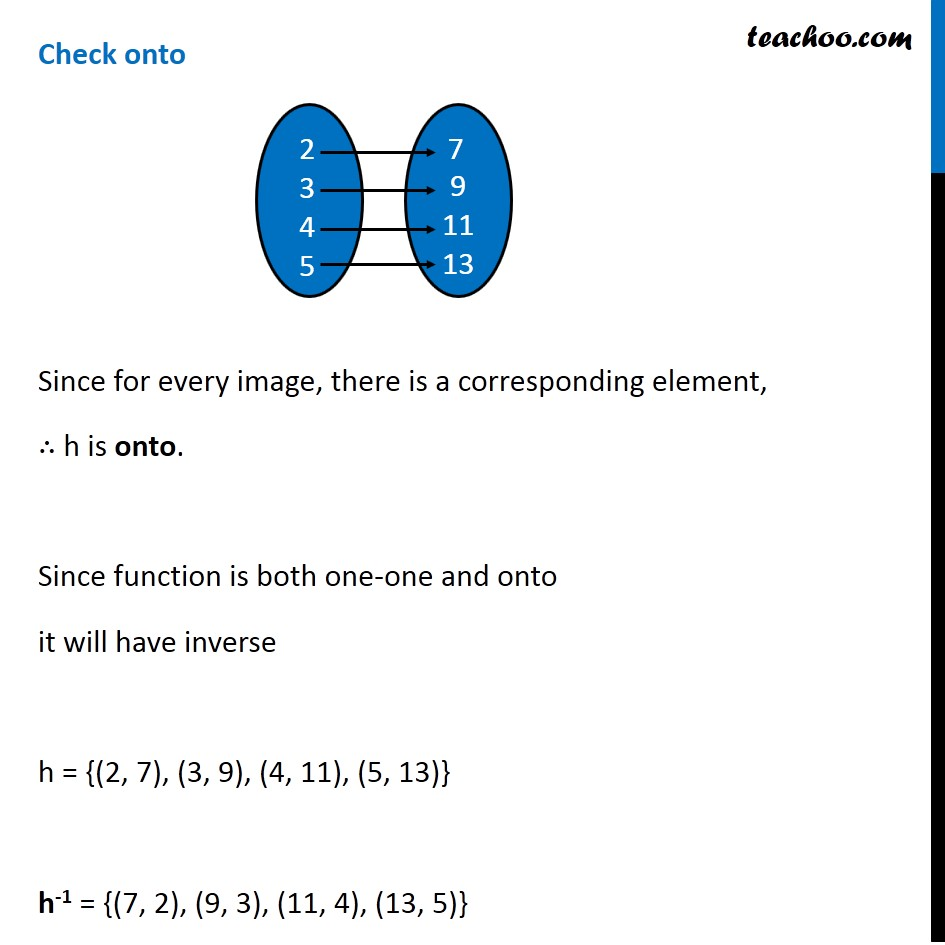Ex 1.3

Chapter 1 Class 12 Relation and Functions
Serial order wiseGet live Maths 1-on-1 Classs - Class 6 to 12

### Transcript

Ex 1.3, 5 State with reason whether following functions have inverse (iii) h: {2, 3, 4, 5} → {7, 9, 11, 13} with h = {(2, 7), (3, 9), (4, 11), (5, 13)} A function has inverse if it is one-one and onto Check one one h = {(2, 7), (3, 9), (4, 11), (5, 13)} Since each element has unique image, h is one-one Check onto Since for every image, there is a corresponding element, ∴ h is onto. Since function is both one-one and onto it will have inverse h = {(2, 7), (3, 9), (4, 11), (5, 13)} h-1 = {(7, 2), (9, 3), (11, 4), (13, 5)}